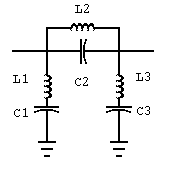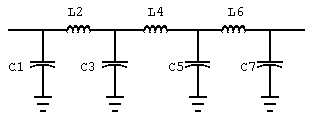# CHEBYSHEV/BUTTERWORTH FILTER DESIGN

Milazzo, Carol F., KP4MD, "Chebyshev/Butterworth Filter Design," unpublished. An IBM BASIC program to design Chebyshev and Butterworth low-pass, high-pass, band-pass and band-stop filters.

## Theory

This program was written in PC-BASIC for automated design of Chebyshev and Butterworth filters in the low-pass, high-pass, band-pass and band-stop configurations. These filters are composed of a series of circuit branches which are alternately connected in series or shunt with the source-to-load path. In low-pass and high-pass filters, each branch is either a capacitor or an inductor. In band-pass and band-stop filters, each branch is either a series or parallel resonant circuit composed of a capacitor and an inductor. The Butterworth design offers a smooth response curve with maximal flatness at zero frequency. The Chebyshev design offers a steeper response curve at the 3 dB cutoff frequency and requires fewer componenets, but it requires much closer tolerance at the design frequency. This program uses the original synthesis formulas to design a normalized filter with equal impedance terminations, and then scales the filter to the desired impedance and frequency.

## Operation

In using the program, the user first specifies the frequency units (Hz, kHz, or MHz) and the type of filter desired. If a low-pass or high-pass filter was specified, two frequencies and their respective attenuations in decibels are required. If a band-pass or band-stop filter was chosen, then a center frequency is first supplied followed by two bandwidth frequencies and their respective attenuations. (The specified attenuation must be greater than zero). In program statements 110-130 the minimum number of branches for the filters is calculated. The value for the Butterworth filter is derived from the Butterowrth response formula. As no corresponding derivation was found for the Chebyshev filter, the number of required branches is estimated to be the next odd number less than that calculated for the Butterworth design. (The design of even-order Chebyshev filters requires much more complicated synthesis formulas and was not attempted in this program). In statement 140 the 3 dB cutoff frequency for the two designs is then calculated. The ripple attenuation of the Chebyshev filter must be specified not greater than 3 decibels. When the filter component values are desired, the termination resistance and the first branch type (shunt or series) must be supplied. The normalized Butterworth filter component values are calculated in statement 270; the Chebyshev values are calculated in statements 300 to 330. These values are scaled to the desired frequency and impedance in the subroutine at statements 340 to 390; and the subroutine at statements 400 to 510 rounds these values to 3 significant digits and prints them in the most common units. The statements following 520 calculate the response curve in the form of a table of attenuation vs. frequency for any specified range and increment of frequency.

Two examples follow to demonstrate the use of this program. First is a bandstop filter with a center frequency of 27.185 MHz, 20 dB attenuation over a 440 kHz bandwidth and a minimum 1 dB attenuation over a 1.63 MHz bandwidth. Both the Butterworth and Chebyshev filters require 3 branches, but the Chebyshev filter has a steeper curve and higher attenuation near the center frequency.

The second example is a low-pass filter with an attenuation of 1 dB at 30 MHz and 30 dB at 54 MHz. The Butterworth design requires 7 components while the Chebyshev design gives a comparable response curve with only 5 components.

## Bandstop Filter Design

Center Frequency 27.185 MHz
```Bandwidth Freq MHz   Attenuation (dB)
.44                  20
1.63                 1

Design Type   Branches
Butterworth   3
Chebyshev     3

```
Chebyshev Ripple (dB): .1
Resistance (ohms): 50

### Butterworth Bandstop Filter Components

Branch 1 Shunt
L 1 = 6.12 µH in Series with
C 1 = 5.6 pF

Branch 2 Series
C 2 = .00122 µF in Parallel with
L 2 = .028 µH

Branch 3 Shunt
L 3 = 6.12 µH in Series with
C 3 = 5.6 pF

### Chebyshev Bandstop Filter Components

Branch 1 Shunt
L 1 = 4.27 µH in Series with
C 1 = 8.030001 pF

Branch 2 Series
C 2 = .00153 µF in Parallel with
L 2 = .0223 µH

Branch 3 Shunt
L 3 = 4.27 µH in Series with
C 3 = 8.030001 pFFigure 1. Schematic diagram of three branch bandstop filter.

### Frequency Response Curve

Frequency Range (MHz)
Lower Limit: 26
Upper Limit: 28
In steps of .1
```MHz    Butterworth     Chebyshev Attenuation (dB)
26     9.999999E-02    .03
26.1   .17             .01
26.2   .31             0
26.3   .58             .11
26.4   1.13            .56
26.5   2.25            1.95
26.6   4.43            5.03
26.7   8.14            10
26.8   13.68           16.57
26.9   21.4            24.98
27     32.68           36.68
27.1   53              57.21
27.2   98.24           102.52
27.3   45.21           49.38
27.4   28.96           32.86
27.5   19.11           22.53
27.6   12.19           14.86
27.7   7.24            8.849999
27.8   3.98            4.39
27.9   2.08            1.71
28     1.08            .51

```

## Lowpass Filter Design

```Freq MHz   Attenuation (dB)
29.7       1
54         30

Design Type   Branches
Butterworth   7
Chebyshev     5

```
Chebyshev Ripple (dB): .1
Resistance (ohms): 50

### Butterworth Lowpass Filter Components

Branch 1 Shunt
C 1 = 43.30001 pF

Branch 2 Series
L 2 = .303 µH

Branch 3 Shunt
C 3 = 175 pF

Branch 4 Series
L 4 = .487 µH

Branch 5 Shunt
C 5 = 175 pF

Branch 6 Series
L 6 = .303 µH

Branch 7 Shunt
C 7 = 43.30001 pFFigure 2. Schematic diagram of seven branch low-pass filter.

Branch 1 Shunt
C 1 = 122 pF

Branch 2 Series
L 2 = .364 µH

Branch 3 Shunt
C 3 = 210 pF

Branch 4 Series
L 4 = .364 µH

Branch 5 Shunt
C 5 = 122 pF

### Frequency Response Curve

Frequency Range (MHz)
Lower Limit: 20
Upper Limit: 60
In steps of 2
```MHz   Butterworth    Chebyshev Attenuation (dB)
20     0             .02
22    .02            .07
24    .06            9.999999E-02
26    .17            .07
28    .47            .01
30    1.13           .11
32    2.39           .9299999
34    4.34           3.01
36    6.84           6.04
38    9.62           9.34
40    12.49          12.53
42    15.33          15.51
44    18.1           18.27
46    20.77          20.83
48    23.34          23.21
50    25.81          25.45
52    28.19          27.55
54    30.48          29.53
56    32.69          31.41
58    34.83          33.21
60    36.89          34.91

```

### BASIC Program Listing filter.bas

10 CLS:PRINT"Chebyshev/Butterworth Filter Design":PRINT:PRINT"by Carol F. Milazzo, KP4MD"
20 IF INKEY\$="" THEN 20
30 DIM A(2),B(2),C(2),F(2),D(256,2),N(2),D\$(2),F\$(3),T\$(4):D\$(1)="Butterworth":D\$(2)="Chebyshev":F\$(1)="Hz":F\$(2)="kHz":F\$(3)="MHz":T\$(1)="Lowpass":T\$(2)="Highpass":T\$(3)="Bandpass":T\$(4)="Bandstop":PI=3.1415926#
40 CLS:PRINT"Chebyshev/Butterworth Filter Design":PRINT:PRINT"Frequency Units":PRINT:PRINT" 0 . Exit":FOR K=1 TO 3:PRINT K;". ";F\$(K):NEXT K:PRINT
50 INPUT"Select: ",FX:IF FX<1 OR FX>3 THEN END
60 F\$=F\$(FX):FP=1000^(FX-1)
70 PRINT:PRINT" 1. ";T\$(1);"/";T\$(2):PRINT" 2. ";T\$(3);"/";T\$(4):PRINT
80 INPUT"Select: ",TY:IF TY<0 OR TY>2 THEN 40
90 CLS:PRINT"Filter Specifications":PRINT:Q=0:B\$="":IF TY=2 THEN Q=1:B\$="Bandwidth ":PRINT"Center Freq.(";F\$;:INPUT"): ",G0:F0=G0*FP:PRINT
100 FOR K=1 TO 2:PRINT K;". ";B\$;"Freq.(";F\$;:INPUT") ",G(K):IF G(K)<=0 THEN RUN 30
110 INPUT" Attn.(dB) ",A(K):IF A(K)<=0 THEN RUN 30
120 F(K)=G(K)*FP:PRINT:NEXT K
130 N=LOG((10^(.1*A(1))-1)/(10^(.1*A(2))-1))/(2*LOG(F(1)/F(2))):S=SGN(N):T=Q+Q+1.5-.5*S:N(1)=-INT(-(ABS(N))):NX=0:IF N(1)/2>INT(N(1)/2) THEN NX=1
140 NY=0:IF N(1)<4 THEN NY=1
150 N(2)=N(1)-1-NX+2*NY:AX=0:IF A(1)>A(2) THEN AX=1
160 FOR K=1 TO 2:C(K)=F(1+AX)*(10^(.1*A(1+AX))-1)^(-.5/S/N(K)):NEXT K
170 CLS:PRINT T\$(T);" Filter Design":PRINT:IF T>2 THEN PRINT"Center Frequency ";G0;" ";F\$:PRINT:PRINT B\$
180 PRINT"Freq ";F\$;" Attenuation (dB)":FOR K=1 TO 2:PRINT G(K),A(K):NEXT K:PRINT:PRINT"Design Type Branches":PRINT D\$(1),N(1):PRINT D\$(2),N(2):PRINT:PRINT"0. New Filter":PRINT"1. Components Values":PRINT"2. Response Curve":PRINT
190 INPUT"Select: ",D:IF D<0 OR D>2 THEN 190
200 IF D=0 THEN 90
210 PRINT:INPUT"Chebyshev Ripple (dB): ",RDB:IF RDB<=0 OR RDB>3 THEN 170
220 Y=RDB/17.37:Y=LOG((EXP(Y)+EXP(-Y))/(EXP(Y)-EXP(-Y)))/2/N(2):Y=(EXP(Y)-EXP(-Y))/2:E=SQR(10^(.1*RDB)-1):C=LOG(1/E+SQR(1/E/E-1))/N(2):C=(EXP(C)+EXP(-C))/2:IF D=2 THEN 540
230 PRINT:INPUT"Resistance (ohms): ",R:IF R<=0 THEN 210
240 PRINT:PRINT"First Element:":PRINT"1. Shunt":PRINT"2. Series":PRINT
250 INPUT"Select: ",V:IF V<1 OR V>2 THEN 230
260 V=V+V-3:U=S*V:PRINT:PRINT"Design Type:":PRINT"1. ";D\$(1):PRINT"2. ";D\$(2):PRINT
270 INPUT"Select: ",D:IF D<1 OR D>2 THEN 240
280 CLS:PRINT D\$(D);" ";T\$(T);" Filter Components":PRINT:IF D=2 THEN 320
290 FOR K=1 TO N(1):G=2*SIN((K+K-1)*PI/2/N(1)):GOSUB 360:PRINT:NEXT K
300 IF INKEY\$="" THEN 300
310 GOTO 170
320 FOR K=1 TO N(2):A=SIN((K+K-1)*PI/2/N(2)):B=Y*Y+(SIN(K*PI/N(2)))^2
330 IF K=1 THEN G=2*A*C/Y
340 IF K>1 THEN G=4*A*C*C*A1/B1/G1
350 GOSUB 360:A1=A:B1=B:G1=G:PRINT:NEXT K:GOTO 300
360 IF V>0 THEN PRINT"Branch ";K;" Series"
370 IF V<0 THEN PRINT"Branch ";K;" Shunt "
380 X=G^S/2/PI/C(D)*R^U:GOSUB 420:V=-V:U=-U:IF Q=0 THEN PRINT:RETURN
390 IF U<0 THEN PRINT " in Series with"
400 IF U>0 THEN PRINT " in Parallel with"
410 X=1/(4*PI*PI*F0*F0*X):GOSUB 420:PRINT:RETURN
420 IF U>0 THEN PRINT " L";K;" = ";
430 IF U<0 THEN PRINT " C";K;" = ";
440 P=INT(LOG(X)/LOG(10)):X1=10^(P-2)*INT(X*10^(2-P)+.5):IF P<0 THEN 460
450 PRINT X1;" ";:GOTO 510
460 IF P<-3 OR U<0 THEN 480
470 PRINT 1000*X1;" m";:GOTO 510
480 IF P<-9 AND U<0 THEN 500
490 PRINT 1000000!*X1;" µ";:GOTO 510
500 PRINT 1E+12*X1;" p";
510 IF U>0 THEN PRINT "H";
520 IF U<0 THEN PRINT "F";
530 RETURN
540 CLS:PRINT"Frequency Response Curve":PRINT:PRINT"Frequency Range (";F\$;")":INPUT"Lower Limit: ",FL:INPUT"Upper Limit: ",FU:INPUT"In steps of ",FS:I=FL*FP:J=FU*FP:K=FS*FP
550 CLS:PRINT" ";F\$;TAB(14);D\$(1);:IF N(2)<23 THEN PRINT TAB(28);D\$(2);
560 PRINT" Attenuation (dB)":FOR F=I TO J STEP K:X=(F-I)/K+1:F1=F:IF Q>0 THEN F1=ABS(F-F0*F0/F)
570 D(X,1)=.01*INT(1000*LOG(1+(F1/C(1))^(2*S*N(1)))/LOG(10)+.5):IF N(2)>21 THEN 720
580 O=C*(F1/C(2))^S:ON N(2) GOTO 600,0,610,0,620,0,630,0,640,0,650,0,660,0,670,0,680,0,690,0,700,0
590 REM Chebyshev polynomials
600 CN=O:GOTO 710
610 CN=4*O^3-3*O:GOTO 710
620 CN=16*O^5-20*O^3+5*O:GOTO 710
630 CN=64*O^7-112*O^5+56*O^3-7*O:GOTO 710
640 CN=256*O^9-576*O^7+432*O^5-120*O^3+9*O:GOTO 710
650 CN=1024*O^11-2816*O^9+2816*O^7-1232*O^5+220*O^3-11*O:GOTO 710
660 CN=4096*O^13-13312*O^11+16640*O^9-9984*O^7+2912*O^5-364*O^3+13*O:GOTO 710
670 CN=16384*O^15-61440!*O^13+92160!*O^11-70400!*O^9+28800*O^7-6048*O^5+560*O^3-15*O:GOTO710
680 CN=65536!*O^17-278528!*O^15+487424!*O^13-452608!*O^11+239360!*O^9-71808!*O^7+11424*O^5-816*O^3+17*O:GOTO710
690 CN=262144!*O^19-1245184!*O^17+2490368!*O^15-2723840!*O^13+1770496!*O^11-695552!*O^9+160512!*O^7-20064*O^5+1140*O^3-19*O:GOTO710
700 CN=1048576!*O^21-5505024!*O^19+1.238631E+07*O^17-1.559757E+07*O^15+1.204224E+07*O^13-5870593!*O^11+1793792!*O^9-329472!*O^7+33264!*O^5-1540*O^3+21*O
710 D(X,2)=.01*INT(1000*LOG(1+E*E*CN*CN)/LOG(10)+.5)
720 PRINT F/FP;TAB(15);D(X,1);:IF N(2)<23 THEN PRINT TAB(30);D(X,2);
730 PRINT:NEXT F:GOTO 300
1000 SCREEN 2:CLS
1010 LINE (64,16)-(64,176)
1020 LINE (64,16)-(608,16)
1030 LINE (608,16)-(608,176)
1040 LINE (64,176)-(608,176)
1050 LOCATE 1,20:PRINT D\$(D);" ";T\$(T);" Filter Frequency Response Curve"
1060 LOCATE 1,1:PRINT"Attenuation"
1070 LOCATE 22,74:PRINT"Freq."